# Area + spherical cap - math problems

#### Number of problems found: 15

• Spherical cap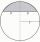What is the surface area of a spherical cap, the base diameter 20 m, height 2 m.
• Spherical cap 4What is the surface area of a spherical cap, the base diameter 20 m, height 2.5 m? Calculate using formula.
• Spherical capPlace a part of the sphere on a 4.6 cm cylinder so that the surface of this section is 20 cm2. Determine the radius r of the sphere from which the spherical cap was cut.
• Sphere - partsCalculate the area of a spherical cap, which is part of an area with base radius ρ = 9 cm and a height v = 3.1 cm.
• The roof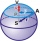The roof has the shape of a spherical canopy with a base diameter of 8 m and a height of 2 m, calculate the area of the foil with which the roof is covered, when we calculate 13% for waste and residues.
• AirplaneAviator sees part of the earth's surface with an area of 200,000 square kilometers. How high he flies?
• PilotHow high is the airplane's pilot to see 0.001 of Earth's surface?
• Above EarthTo what height must a boy be raised above the earth in order to see one-fifth of its surface.
• Tropical, mild and arctic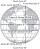How many percents of the Earth's surface lies in the tropical, mild and arctic range? The border between the ranges is the parallel 23°27 'and 66°33'.
• Hemisphere - roof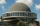The shape of the observatory dome is close to the hemisphere. Its outer diameter is 11 m. How many kilograms of paint and how many liters of thinner is used for its double coat if you know that 1 kg of paint diluted with 1 deciliter of thinner will paint
• Two hemispheres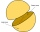In a wooden hemisphere with a radius r = 1, a hemispherical depression with a radius r/2 was created so that the bases of both hemispheres lie in the same plane. What is the surface of the created body (including the surface of the depression)?
• The observatoryThe dome of the hemisphere-shaped observatory is 5.4 meters high. How many square meters of sheet metal needs to be covered to cover it, and 15 percent must be added to the minimum amount due to joints and waste?
• Sphere parts, segmentA sphere with a diameter of 20.6 cm, the cut is a circle with a diameter of 16.2 cm. .What are the volume of the segment and the surface of the segment?
• Tropics and polar zones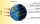What percentage of the Earth’s surface lies in the tropical, temperate and polar zone? Individual zones are bordered by tropics 23°27' and polar circles 66°33'
• The cylindrical container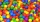The cylindrical container has a base area of 300 cm3 and a height of 10 cm. It is 90% filled with water. We gradually insert metal balls into the water, each with a volume of 20 cm3. After inserting how many balls for the first time does water flow over

Do you have an interesting mathematical word problem that you can't solve it? Submit a math problem, and we can try to solve it.

We will send a solution to your e-mail address. Solved examples are also published here. Please enter the e-mail correctly and check whether you don't have a full mailbox.

Please do not submit problems from current active competitions such as Mathematical Olympiad, correspondence seminars etc...

Area - math word problems. Spherical cap Problems.